### IMO Shortlist 1960 problem 5

Kvaliteta:
Avg: 0,0
Težina:
Avg: 0,0
Consider the cube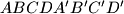$ABCDA'B'C'D'$ (with face$ABCD$ directly above face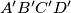$A'B'C'D'$).

a) Find the locus of the midpoints of the segments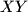$XY$, where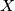$X$ is any point of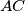$AC$ and$Y$ is any piont of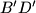$B'D'$;

b) Find the locus of points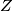$Z$ which lie on the segment$XY$ of part a) with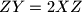$ZY=2XZ$.
Izvor: Međunarodna matematička olimpijada, shortlist 1960